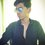# GCD propertyQuestions asks to confirm the property.Note by Shivamani Patil
6 years, 7 months ago

This discussion board is a place to discuss our Daily Challenges and the math and science related to those challenges. Explanations are more than just a solution — they should explain the steps and thinking strategies that you used to obtain the solution. Comments should further the discussion of math and science.

When posting on Brilliant:

• Use the emojis to react to an explanation, whether you're congratulating a job well done , or just really confused .
• Ask specific questions about the challenge or the steps in somebody's explanation. Well-posed questions can add a lot to the discussion, but posting "I don't understand!" doesn't help anyone.
• Try to contribute something new to the discussion, whether it is an extension, generalization or other idea related to the challenge.

MarkdownAppears as
*italics* or _italics_ italics
**bold** or __bold__ bold
- bulleted- list
• bulleted
• list
1. numbered2. list
1. numbered
2. list
Note: you must add a full line of space before and after lists for them to show up correctly
paragraph 1paragraph 2

paragraph 1

paragraph 2

[example link](https://brilliant.org)example link
> This is a quote
This is a quote
    # I indented these lines
# 4 spaces, and now they show
# up as a code block.

print "hello world"
# I indented these lines
# 4 spaces, and now they show
# up as a code block.

print "hello world"
MathAppears as
Remember to wrap math in $$ ... $$ or $ ... $ to ensure proper formatting.
2 \times 3 $2 \times 3$
2^{34} $2^{34}$
a_{i-1} $a_{i-1}$
\frac{2}{3} $\frac{2}{3}$
\sqrt{2} $\sqrt{2}$
\sum_{i=1}^3 $\sum_{i=1}^3$
\sin \theta $\sin \theta$
\boxed{123} $\boxed{123}$

Sort by:

$c | (a+b) \rightarrow c|a \wedge c|b$ is wrong. Easily counterexample as $a = 4, b = 5, c = 3)$.

- 6 years, 7 months ago

Hint: use Bezout's identity

- 6 years, 7 months ago

Another hint: $(a,b) = 1$ if and only if $ax+by = 1$.

- 6 years, 7 months ago

Hey,@Samuraiwarm Tsunayoshi check my sol in Kartik Sharma's solution's replay box.

- 6 years, 7 months ago

Let $a = {{p}_{1}}^{{e}_{1}}{{p}_{2}}^{{e}_{2}}{{p}_{3}}^{{e}_{3}}..... {{p}_{n}}^{{e}_{n}}$

Now, $a = 0 mod {p}_{i}$

We know that gcd(a,b) = 1, so none of the ${p}_{i}$ divide b

$cn = a + b$

$cn mod {p}_{1} = 0 + x$

$cn = x mod {p}_{1}$

And so on, till ${p}_{n}$ which tells us that ${p}_{i}$ doesn't divide c. Therefore there is no common divisor of c and a, so, $gcd(a,c) = 1$

Similarly we can show for b.

@shivamani patil Check out and now I think it is fine.

- 6 years, 7 months ago

If gcd(a,b) then $ax+by=1$ also $cn=a+b$ for some $n$ because $c|a+b$.Therefore $cn-b=a$,substituting $cn-b=a$ in first equation we get $x(cn-b)+by=1=cnx-bx+by=c(nx)+b(y-x)$ which implies gcd(c,b)=1.Similarly gcd(c,a)=1.@Kartik Sharma how is it??Your's is good.

- 6 years, 7 months ago

Yep, looks good.

- 6 years, 7 months ago

- 6 years, 7 months ago

But I solved it independently @Kartik Sharma

- 6 years, 7 months ago

Note that $a|b$ implies that there exists an integer $k$ suck that $ka = b$, not $kb = a$. So your assumption that $c = n(a+b)$ is wrong. It should be $cn = a+b$.

- 6 years, 7 months ago

Oh damn yes ! I knew it but just a silly mistake. Now, I am gonna edit it.

- 6 years, 7 months ago